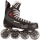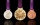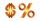# Competitors

In the first round of slalom fell 15% of all competitors and in the second round another 10 racers. Together, 40% of all competitors fell. What was the total number of competitors?

Result

n =  40

#### Solution:

0.15n + 10 = 0.40n

0.25n = 10

n = 40

Calculated by our simple equation calculator.Our examples were largely sent or created by pupils and students themselves. Therefore, we would be pleased if you could send us any errors you found, spelling mistakes, or rephasing the example. Thank you!

Leave us a comment of this math problem and its solution (i.e. if it is still somewhat unclear...):Be the first to comment!Tips to related online calculators
Do you have a linear equation or system of equations and looking for its solution? Or do you have quadratic equation?

## Next similar math problems:

1. Ice skatesIce skates were raised twice, the first time by 25%, the second time by 10%. After the second price, their cost was 82.5 euros. What was the original price of skates?
2. GirlsThe children's competition was attended by 63 girls, which is 30% of all children's participants. How many children attended this competition?
3. The Chemistry testThe Chemistry test contained 8 questions, each with 3 points. Peter scored 21 points. How many percent did Peter write a test?
4. RewardJanko and Peter divided the reward from the brigade so that Peter got 5/8 of the reward. What percentage of Janko's reward got?
5. Unknown number15% of the unknown number is 18 less than 21% of the same number. What is the unknown number?
6. PersonsPersons surveyed:100 with result: Volleyball=15% Baseball=9% Sepak Takraw=8% Pingpong=8% Basketball=60% Find the average how many like Basketball and Volleyball. Please show your solution.
7. PupilsThere are 350 girls in the school, and the other 30% of the total number of pupils are boys. How many pupils does the school have?
8. SummerjobThe temporary workers planted new trees. Of the total number of 500 seedlings, they managed to plant 426. How many percents did they meet the daily planting limit?
9. Art schoolEvery fifth pupil 9A goes to art school. How many percent of pupils in class 9A go to art school?
10. Percentages52 is what percent of 93?
11. PercentsHow many percents is 900 greater than the number 750?
12. The ballThe ball was discounted by 10 percent and then again by 30 percent. How many percent of the original price is now?
13. Fifth of the numberThe fifth of the number is by 24 less than that number. What is the number?
14. Perctentages35% of what number is 35?
15. Profit gainIf 5% more is gained by selling an article for Rs. 350 than by selling it for Rs. 340, the cost of the article is:
16. Sale offThe TV went down 10% and then 10% off the original price again. Now it costs 300 €. What was its original price?
17. Reducing numberReducing the an unknown number by 28.5% we get number 243.1. Determine unknown number.